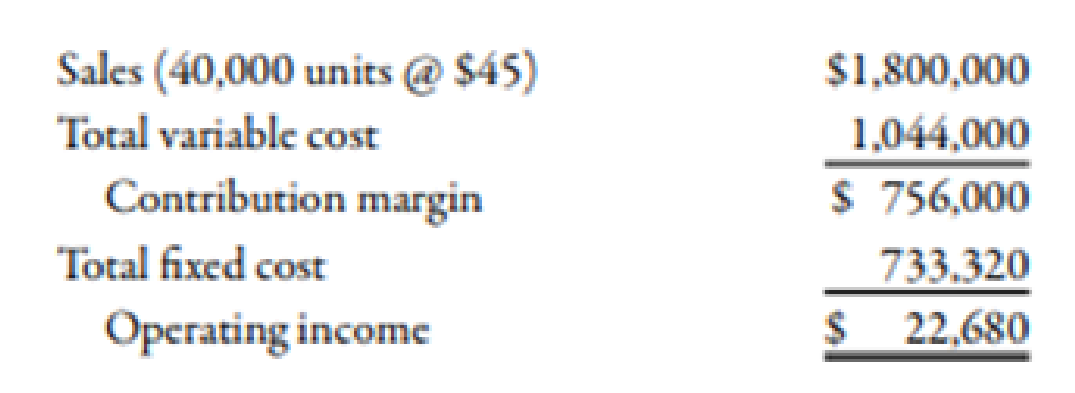Chapter 7, Problem 48E### Managerial Accounting: The Corners...

7th Edition
Maryanne M. Mowen + 2 others
ISBN: 9781337115773

#### Solutions

Chapter
Section### Managerial Accounting: The Corners...

7th Edition
Maryanne M. Mowen + 2 others
ISBN: 9781337115773
Textbook Problem
39 views

# Margin of Safety and Operating LeverageMedina Company produces a single product. The projected income statement for the coming year is as follows:(Note: Round all dollar answers to the nearest dollar. Round contribution margin ratio and degree of operating leverage to two decimal places.)Required: 1. Compute the break-even sales dollars. 2. Compute the margin of safety in sales dollars. 3. Compute the degree of operating leverage. (Note: Round answer to two decimal places.) 4. Compute the new operating income if sales are 20% higher than expected. (Note: Round answer to the nearest dollar.)

1.

To determine

Compute the break-even sales dollars.

Explanation

Break-Even Point:

The point or situation where the amount of total cost is equivalent to total revenue is known as the break-even point. It is the point where there is no loss or no profit.

Use the following formula to calculate the break-even sales dollars:

Break-even sales dollars=Fixed costsContribution margin ratio1

Substitute $733,320 for fixed costs and 42% for contribution margin ratio in the above formula. Break-even sales dollars=$733,32042%

2.

To determine

Compute margin of safety in sales dollars.

3.

To determine

Compute the degree of operating leverage.

4.

To determine

Compute the amount of new operating income if the sales are increased by 20%.

### Still sussing out bartleby?

Check out a sample textbook solution.

See a sample solution

#### The Solution to Your Study Problems

Bartleby provides explanations to thousands of textbook problems written by our experts, many with advanced degrees!

Get Started

#### Find more solutions based on key concepts